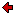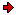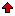Journal of Technical
Physics

A QUARTERLY JOURNALPrevious issue vol. 42, no. 2 (2001) vol. 42, no. 3 (2001)Next issuevol. 42, no. 4 (2001)

### Contents of issue 3, vol. 42

1. Z.T. Kurlandzka: Irwin criterion for dielectric with strong electrostriction
2. R. Brojewski: Structural equivalence and stability of some atmospheric air flow models
3. J. Avsec and M. Marčič: Calculation of thermophysical properties for solids with application of statistical thermodynamics
4. A. Pielorz and W. Nadolski: Dynamic analysis of nonlinear loads on gear teeth in discrete-continuous model of single gear transmission
5. J. Warmiński: Influence of external force on the parametric and self-excited system

#### Brief Notes

6. Cz. Rymarz: Ponderomotive force in laboratory and co-moving frames of reference at the CGS and SI system of dimensional units
7. J. Janucki and J. Owsik: Solution of a problem of diffraction from a plane grating at normal wave incidence
8. J. Janucki and J. Owsik: Diffraction from rectangular-profile gratings

Z.T. Kurlandzka: Irwin criterion for dielectric with strong electrostriction
A fracture criterion, being an extension of the Irwin criterion for the case of elastic dielectric with strong electrostriction, is derived. The paper is a generalization of the previous paper by the author, where electromagnetic interactions resulting from the Lorentz force according to the Dixon-Eringen model were taken into account. Here nonlinear electromagnetic interactions are supplemented with terms, which include the additional material constants responsible for strong electrostriction.

ContentsR. Brojewski: Structural equivalence and stability of some atmospheric air flow models
Two ways of modeling of flows in lower atmosphere forced by stationary flows in upper layers of the atmosphere are presented in the paper. Attention is paid to inequivalence, due to the Coriolis force, of differential models based on 3-D equations of flow of liquid and based on the flow description by means of the stream function. Numerical difficulties of the two ways of solving the flow problem and a way of overcoming them are presented. Structural instability of the models is proved and some of the responsible factors are indicated. Among others are also formal parameters of the model, e.g. the time step of integration.

ContentsJ. Avsec and M. Marčič: Calculation of thermophysical properties for solids with application of statistical thermodynamics
The paper deals with a mathematical model for calculation of the thermodynamic properties of solids. The mathematical model, based upon statistical thermodynamics, is designed to assess the impact of atom vibration, electron excitation and the effect of intermolecular energy between atoms in a crystal. To calculate the configuration integral, the perturbation theory was used with the van der Waals model as perturbation. The temperature-variable coefficients were introduced into the model presented in this paper. Finally, the model was compared with the experimental data proving a good matching.

ContentsA. Pielorz and W. Nadolski: Dynamic analysis of nonlinear loads on gear teeth in discrete-continuous model of single gear transmission
In the paper, four nonlinear functions describing forces occurring between the teeth in a single gear transmission are discussed. It is assumed that gear teeth can have characteristics of a soft as well as of a hard type. In the study, a discrete-continuous model consisting of two ponderable shafts torsionally deformed and four rigid bodies is used. Considerations are performed by means of the wave method which enables to determine nonlinear dynamic loads in steady as well as in transient states.

ContentsJ. Warmiński: Influence of external force on the parametric and self-excited system
Influence of an external harmonic force on a non-linear parametrically and self-excited system with two degrees of freedom has been investigated in this paper. Vibration analysis has been carried out for coupled Rayleigh-Mathieu-Duffing oscillators. By means of the multiple scales' method, the existence and stability of periodic and quasi-periodic solutions close to the main parametric resonances have been considered. Influence of a small external force on vibrations in the synchronization, chaotic and hyperchaotic region has been analyzed. Transition from regular vibrations to chaotic and hyperchaotic motion has been presented as well.

ContentsCz. Rymarz: Ponderomotive force in laboratory and co-moving frames of reference at the CGS and SI system of dimensional units
In this paper, the influence of the system of dimensional units on the uniqueness of the ponderomotive force has been considered. It was established that at the CGS Gauss system, the ponderomotive force is non-unique. The non-uniqueness follows from the so-called dimensional degeneration, what means that several values have the same dimension. Since all the electromagnetic fields, at the CGS Gauss system, have the same dimension, therefore any pair of them can be treated as the density of electromagnetic momentum. In the SI system of units, due to the lower (dimensional) symmetry, the dimensional degeneration disappears and only the pair of inductions D, B has the proper dimension for a ponderomotive force. Therefore the CGS Gauss system of units should not be used in the theory of combined electro-mechanical fields. The forms of ponderomotive force have been formulated in the laboratory and co-moving frames of reference at the Gauss and SI systems of units. It has been also proved that the ponderomotive force is invariant under the change of the frame of reference.

ContentsJ. Janucki and J. Owsik: Solution of a problem of diffraction from a plane grating at normal wave incidence
Periodic structures have been used in many applications of laser technology and radio engineering. They replace the transducers of radiation parameters. The methods employed for calculation of their electrodynamic properties are well known from the literature. However, complicated numerical computations are required to solve the diffraction equations in theirs general form. The paper presents the method of calculation of electrodynamical properties of a plane periodic structure for the particular case of normal wave falling. The used transformations allow to reduce the time of numerical calculations.

ContentsJ. Janucki and J. Owsik: Diffraction from rectangular-profile gratings
Relations describing diffraction of E- and H-polarized electromagnetic waves from rectangular-profile diffraction grating have been formulated. The method of the analysis enables the calculation of diffraction efficiency of single-layer or multilayer gratings, in which the profile of a single period consists of an arbitrary number of perfectly conducting mutually perpendicular surfaces. An algorithm of numerical solution of the equations has been verified by comparing the results obtained by the presented method and the results obtained from the exact analytical solution.

ContentsReprints of the full papers may be obtained from their authors. Contact Editorial Office in case you need the address of the respective author.
 Home Editors Scope Abstracts For authors Subscription

Designed & maintained by Tomasz G. Zieliński.